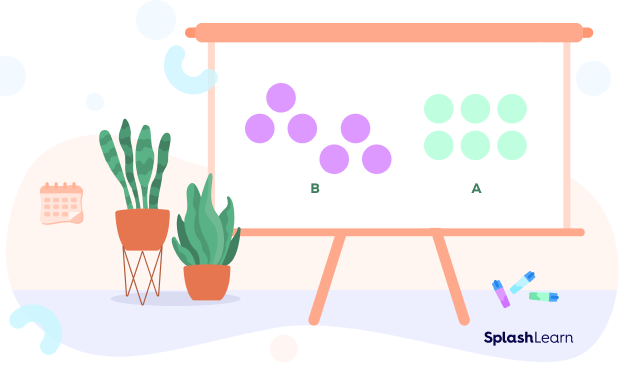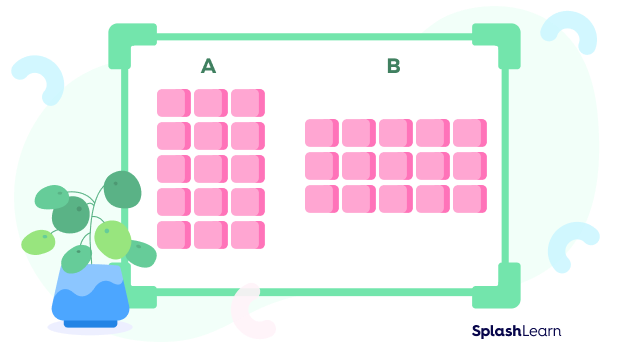# Multiplication Array – Definition with Examples

Home » Math-Vocabluary » Multiplication Array – Definition with Examples

## What is a Multiplication Array?

To understand the concept of multiplication array, let’s first break the concept into two words and understand them separately.

Multiplication is the concept of mathematics that allows us to multiply one number with another. For example, 3 x 5 is three times five, which is 15.

An array is a table-like arrangement representing numbers or data in the form of columns and rows.

A multiplication array creates an arrangement where we display data in the form of an array to find the product of any multiplication question. The array is usually created using small objects or shapes or dots to make it interactive.

For example, to multiply 3 x 5, the same example as above, we will create the following multiplication array.

Tip: While you can use any number of objects in a row or column, use one method for every problem in the beginning to avoid confusion. So, we will represent the first number in the form of rows and the second in the form of columns.

In the above multiplication array, we can find the answer by counting the number of circles, which is 15.

## How Do We Represent a Multiplication Array?

Let’s use the example of 2 x 7, also referred to as 2 by 7.

This means that we have 2 rows and 7 columns.

In this array, every row and column has an equal number of objects.

Another way of looking at it is that we have equal groups in the form of rows or columns. When you take these equal groups and arrange them as columns or rows, we get arrays.

For example, equal groups of two arranged in columns created the above array of stars.

## What are Factors and Products in a Multiplication Array?

We use specific terms for numbers involved in multiplication:

• Factors are the numbers being multiplied
• Product is the result gained from the activity, which is the multiplication

What happens if we change the order of factors in the multiplication equation?

In the above example,  we had 2 rows and 7 columns. What if we make 2 columns and 7 rows?

The answer remains the same; it is still 14.

The situation is identical whether you keep the first number as a row or column, and this is true for every mathematical equation.

If more than two factors are involved in the problem, we can change the order but still receive the exact same answer. This is referred to as Commutative Multiplication Property.

## Solved Examples

Example 1: We need to create a multiplication array for 5 by 6. How will we create it?

Let’s take 5 as rows and 6 as columns.

This means we need to make 5 rows horizontally and 6 columns vertically.

When you count these objects, you will get the following:

5 x 6 = 30

Example 2: Find out the multiplication factors in the given multiplication array:

First, we count the number of rows: 2

Then, we count the number of columns: 4

So, the multiplication factors are 2 x 4, which can also be written as 4 x 2.

Example 3: Find the answer or product for the given multiplication array:

First, we will count the objects across each row, which will give us the product = 12.

If we also need to find the factors, then we count the rows (3) and columns (4).

3 x 4 = 12

## Practice Problems

1

### Which of the following figures is a multiplication array?A
B
Both A and B
None of the above
CorrectIncorrect
A forms an array, but B doesn’t form an array. It helps count and find the answer, but it is forming another shape like a triangle, not an array.
2

### How will we create a multiplication array for the following equation? 3 x 5A
B
Both A and B
None of the above
CorrectIncorrect
Correct answer is: Both A and B
Both A and B represent the multiplication array for the problem (Commutative Multiplication Property)
3

### Find the product for the given multiplication array:11
9
12
15
CorrectIncorrect